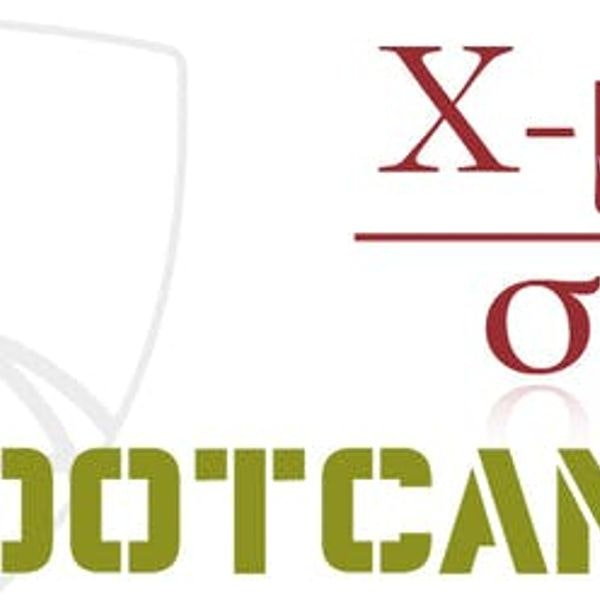# Advanced Statistics for Data Science

Johns Hopkins University

Fundamental concepts in probability, statistics and linear models are primary building blocks for data science work. Learners aspiring to become biostatisticians and data scientists will benefit from the foundational knowledge being offered in this specialization. It will enable the learner to understand the behind-the-scenes mechanism of key modeling tools in data science, like least squares and linear regression.\n\nThis specialization starts with Mathematical Statistics bootcamps, specifically concepts and methods used in biostatistics applications. These range from probability, distribution, and likelihood concepts to hypothesis testing and case-control sampling.\n\nThis specialization also linear models for data science, starting from understanding least squares from a linear algebraic and mathematical perspective, to statistical linear models, including multivariate regression using the R programming language. These courses will give learners a firm foundation in the linear algebraic treatment of regression modeling, which will greatly augment applied data scientists' general understanding of regression models.\n\nThis specialization requires a fair amount of mathematical sophistication. Basic calculus and linear algebra are required to engage in the content.

Certificate Available ✔#### Precalculus through Data and Modelling

Johns Hopkins University

This specialization helps to build the foundational material to use mathematics as a tool to model, understand, and interpret the world around us. This is done through...

#### Fibonacci Numbers and the Golden Ratio

The Hong Kong University of Science and Technology

Learn the mathematics behind the Fibonacci numbers, the golden ratio, and their relationship to each other. These topics may not be taught as part of a typical math...